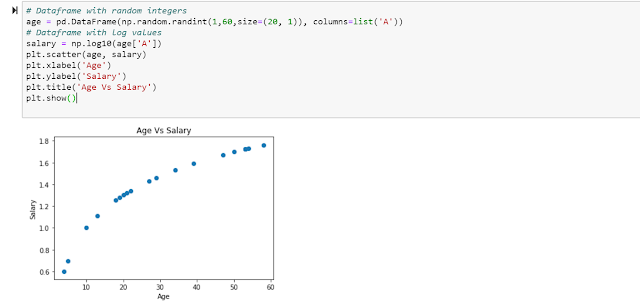## Posts

Showing posts from August, 2019

### Quick Introduction to MatplotlibOverview:In Datascience and Machine learning, plots and graphs play a very important role. To understand the data and its characteristics, it is very important to plot the data and make sense out of it. Matplotlib is a powerful Python library which is very handy in building effective plots. We will take a look at a brief intro to Matplotlib.
Scatter Plot:Scatter Plot plots two variables one each along X and Y axis and provides a view of the relationship or correlation between the two variables. Let us create two variables, where one variable has an exponential relationship with the other (i.e logarithmic relationship).
Let us hypothetically assume that age and salary are related exponentially. So as age increases, salary increases exponentially. Let us create two dataframes with this in mind and plot them. Note how we added labels for X and Y axis and also a title for the plot.

Line Plot:We can also plot two variables and draw a line to reflect the relationship between them. Matplotlib w…

### Quick Introduction to Pandas LibraryOverview:Pandas is a python library which provides very powerful tools to perform complex data manipulations and analysis. In this article, few of the commonly used operations are explained.
A common datastructure is a 'Dataframe'. Dataframe is a matrix type of datastructure with easy access to rows and columns.
Creation of Dataframe:

Accessing data from Dataframe:Data in Dataframe could be accessed in various ways.

Data can also be accessed by slicing the dataframe instead of loops. It is the most efficient way of accessing data.

Creating Dataframe with Index:We can create Dataframe with an index. Let us create a dataframe with date as index.

As we can see from above, the values are filled as NaN (Not a Number). We can replace NaN with zero using fillna() function.

### Quick Introduction to Numpy LibraryOverview:Numpy is a popular library in Python, which is used in Machine Learning and Statistical analysis. This article provides some of the commonly used operations in Numpy. Numpy array operations are efficient and fast compared to normal array operations.

Jupyter Notebook:The code is run using Jupyter Notebook and the relevant snapshots are provided. Note: Numpy could be imported as below:import numpy as np
Array Operations:Basic Operations:Array could be created using np.array(). Shape property provides a tuple of number of rows and columns. We can apply various mathematics functions like mean, median etc on the array.

Comparison in Array: We can apply various techniques to compare one row against other, which would be required when we perform computational analysis.

Array Data Retrieval: We can retrieve data from an array, in terms of rows, columns. We can also, retrieve specific rows or columns or exclude specific rows or columns.

Max Min Operations: We can find out various maximum and m…